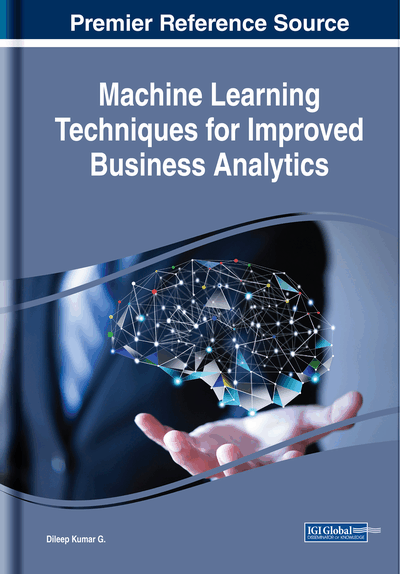# Tree-Based Modeling Techniques

Dileep Kumar G. (Adama Science and Technology University, Ethiopia)
DOI: 10.4018/978-1-5225-3534-8.ch001

## Abstract

Tree-based learning techniques are considered to be one of the best and most used supervised learning methods. Tree-based methods empower predictive models with high accuracy, stability, and ease of interpretation. Unlike linear models, they map non-linear relationships pretty well. These methods are adaptable at solving any kind of problem at hand (classification or regression). Methods like decision trees, random forest, gradient boosting are being widely used in all kinds of machine learning and data science problems. Hence, for every data analyst, it is important to learn these algorithms and use them for modeling. This chapter guide the learner to learn tree-based modeling techniques from scratch.
Chapter Preview
Top

## Decision Tree

Decision tree is a type of supervised learning algorithm (having a predefined target variable) that is mostly used in classification problems (James, Witten, Hastie, & Tibshirani, 2013). It works for both categorical and continuous input and output variables. In this technique, we split the population or sample into two or more homogeneous sets (or sub-populations) based on most significant splitter / differentiator in input variables.

• Example 1: We have a sample of 30 students in a class with three variables Gender (Boy/ Girl), Class (IX/X) and Height (5 to 6 ft). 15 out of these 30 play football in leisure time. Now, create create a model to predict who will play cricket during leisure period? In this problem, we need to segregate students who play football in their leisure time based on highly significant input variable among all three.

This is where decision tree helps, it will segregate the students based on all values of three variable and identify the variable, which creates the best homogeneous sets of students (which are heterogeneous to each other). In Figure 2, 3, and 4, you can see that variable Gender is able to identify best homogeneous sets compared to the other two variables.

As mentioned above, decision tree identifies the most significant variable and its value that gives best homogeneous sets of population.

Let’s look at the commonly used terms used with Decision trees:

• Root Node: It represents entire population or sample and this further gets divided into two or more homogeneous sets.

• Splitting: It is a process of dividing a node into two or more sub-nodes.

• Decision Node: When a sub-node splits into further sub-nodes, then it is called decision node.

• Leaf/Terminal Node: Nodes do not split is called Leaf or Terminal node.

• Pruning: When we remove sub-nodes of a decision node, this process is called pruning. You can say opposite process of splitting.

• Branch/Sub-Tree: A sub section of entire tree is called branch or sub-tree.

• Parent and Child Node: A node, which is divided into sub-nodes is called parent node of sub-nodes whereas sub-nodes are the child of parent node. For example, A is parent node B and C in Figure 1.

## Complete Chapter List

Search this Book:
Reset